ISSN ONLINE(2319-8753)PRINT(2347-6710)

All submissions of the EM system will be redirected to Online Manuscript Submission System. Authors are requested to submit articles directly to Online Manuscript Submission System of respective journal.

# A Novel Control Algorithm for Conversion of AC-DC with Improved Power Factor and Efficiency

 Ch.Sumalatha 1, M.Sreenivasulu Reddy 2 P.G. Scholar, Department Of Electrical Engineering, SIETK, Puttur, A.P., India Assistant Professor, Department Of Electrical Engineering, SIETK, Puttur, A.P., India Related article at Pubmed, Scholar Google

Visit for more related articles at International Journal of Innovative Research in Science, Engineering and Technology

## Abstract

A Single power-conversion ac–dc converter with high power factor and high efficiency. The proposed converter is derived by integrating a full-bridge diode rectifier and a series-resonant active-clamp dc–dc converter. To obtain a high power factor without a power factor correction circuit, this paper proposes a novel control algorithm. The proposed converter provides single power-conversion by using the novel control algorithm for both power factor correction and output control. Also, the active-clamp circuit clamps the surge voltage of switches and recycles the energy stored in the leakage inductance of the transformer. Moreover, it provides zero-voltage turn-on switching of the switches. Also, a series-resonant circuit of the output-voltage doubler removes the reverse-recovery problem of the output diodes. The proposed converter provides maximum power factor 0.995 and maximum efficiency of 95.1% at the full load.

### Keywords

Active-clamp circuit, series-resonant circuit, single power-conversion.

### INTRODUCTION

GENERALLY, the ac–dc converter consists of a full-bridge diode rectifier, a dc-link capacitor and a high frequency dc–dc converter. These converters absorb energy from the ac line only when the rectified line voltage is higher than the dc link voltage. Therefore, these kinds of converters have a highly distorted input current, resulting in a large amount of harmonics and a low power factor. To solve the harmonic pollution caused by ac–dc converters, a number of power factor correction (PFC) ac–dc converters have been proposed and developed- . The PFC ac–dc converter can be implemented by using two power-processing stages. The PFC input stage is used to obtain high power factor while maintaining a constant dc-link voltage. Most PFC circuits employ the boost converter . The output stage, which is a high frequency dc–dc converter, gives a desired output. Two power-processing stages require each control circuit consisting of gate drivers and those controllers. In general, the PFC ac–dc converter can be categorized into two types: two-stage ac–dc converters and single stage ac–dc converters. Two-stage ac–dc converters consist of two powerprocessing stages with their respective control circuits. However, two-stage ac–dc converters raise power losses and the manufacturing cost, eventually reducing the system efficiency and the price competitiveness. In efforts to reduce the component count, the size, and the cost, a number of single-stage ac–dc converters have been proposed and developed. The main idea is that a PFC input stage and a high frequency dc–dc converter are simplified by sharing common switches so that the PFC controller, the PFC switch, and its gate driver can be eliminated. .

### II.CONVENTIONAL METHOD

Fig. 1(a) shows the schematic diagram of the conventional two-stage ac–dc converter. It comprises a full-bridge diode rectifier, a PFC circuit, a control circuit for the PFC circuit, a high frequency dc–dc converter, and a control circuit for output control. The control circuit is composed of gate-drivers and a controller. Namely, two-stage ac–dc converters have two power processing stages with their respective control circuits. Also, the boost type PFC converter used in most PFC input stages requires the dc-link electrolytic capacitor and the inductor. Two control circuits, the dc-link capacitor and the inductor raise the size, weight and the cost of the converter and reduce the price competitiveness. On the other hand, the advantage is to decouple control of the dc-link capacitor voltage from that of the output voltage and realize much tighter output control.However, the single stage ac-dc converters have several disadvantages.First, the power factor is also related to the controller, indicating that the variation of the load or the input voltage will change the power factor. Second, the output voltage control bandwidth is limited to a few hertz not to excessively distort the input current. Third, single-stage ac–dc converters require the dc-link electrolytic capacitor and the inductor for the PFC circuit, just like two-stage converters. Finally, the conventional single-stage ac–dc converters have highvoltage stresses or low power factor.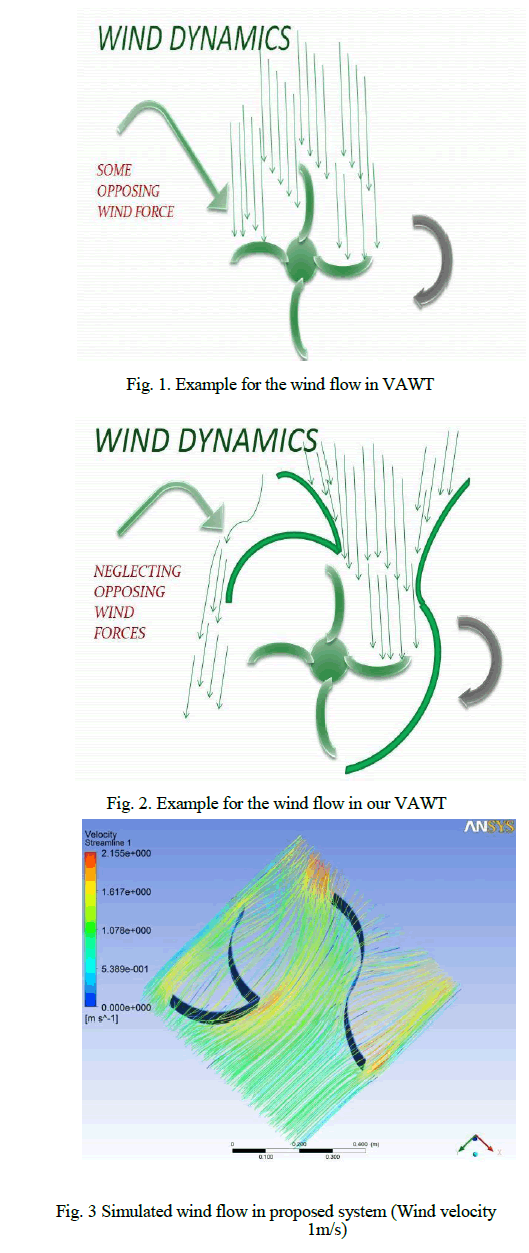### III. PROPOSED METHOD

Fig.2. shows the schematic diagram of the single power-conversion ac–dc converter. It consists of a fullbridge diode rectifier, a high frequency dc–dc converter, and a control circuit. That is, the single power-conversion ac– dc converter has also one control circuit because it has no PFC circuit. However, it requires the control algorithm for both PFC and output control, unlike single-stage ac–dc converters. Also, it has a large ac second-harmonic ripple component reflected at the output voltage in comparison with two-stage and single stage converters because it has no dc-link electrolytic capacitor. However, the single power-conversion ac–dc converter provides a simple structure, a low cost, and low voltage stresses because it has no PFC circuit composed of the inductor, power switching devices and the dc-link electrolytic capacitor. Therefore, the single power-conversion ac–dc converter is preferred option when the cost per unit is more important concerns than reliability.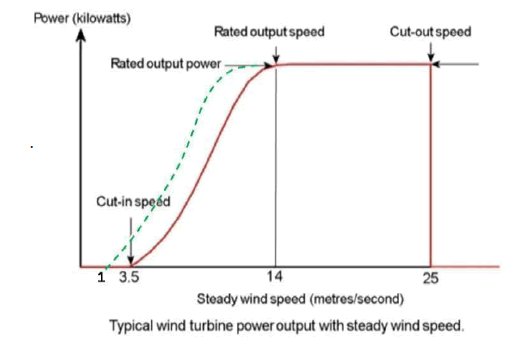The operating modes of the input side and the output side are shown in Figs. 4 and 5, respectively. The rectified input voltage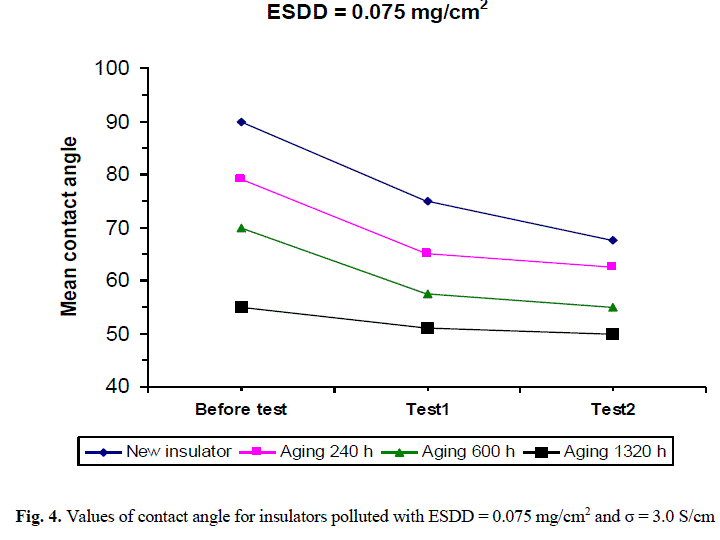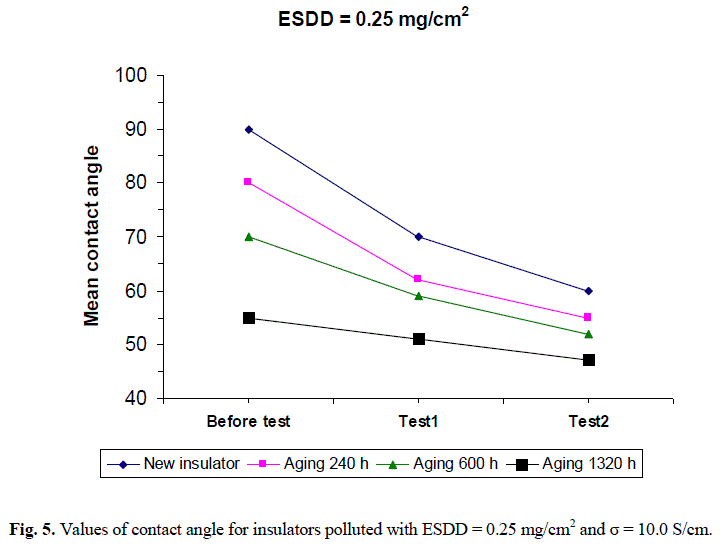### IV. CONTROL ALGORITHM

The proposed converter has no PFC circuit. Therefore, to obtain a high power factor, it requires the control algorithm for both PFC and output control. The duty ratio D according to the input current iin is hard to control because the relation of D and iin is nonlinear. To achieve good controllability, the nonlinear system needs to be transformed into the linear system by the feedback linearization.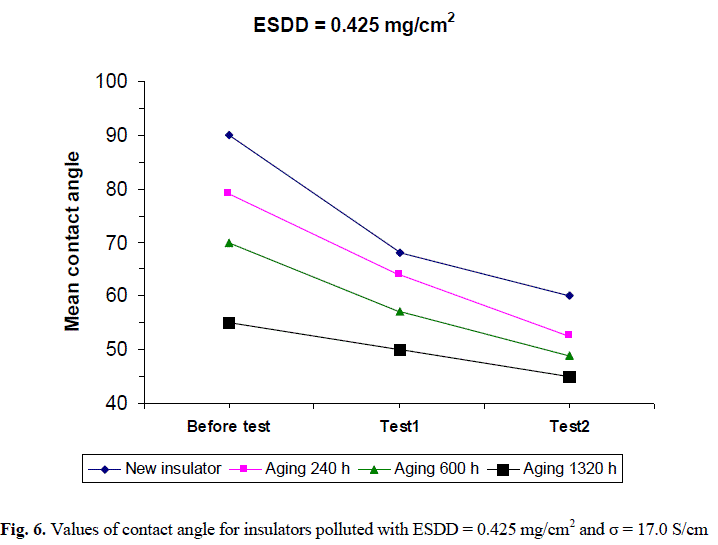Fig. 6 shows the control block diagram of the proposed converter. The voltage controller attempts to minimize the error value as the difference between Vo ,ref and the measured output voltage by adjusting ï¿½ï¿½Ë³ ∗that is, iË³*is calculated by the voltage controller, and then is calculated by the PFC rule in Fig. 6. In order to realize the PFC rule, synchronization with input voltage vin is necessary. Since Vi includes the information about the amplitude and the phase of vin , the synchronization with vin is implemented by using Vi as shown in Fig. 2.
The current controller attempts to , iË³*minimize the error value as the difference betweenand the measured output current io by adjustingΔD. Finally, D is obtained by adding ΔD to Dn . The proposed control system consists of the inner loop and the outer loop. The inner loop is the current control loop and the outer loop is the output voltage control loop. The proposed control system is analyzed by using a small signal model. The crossover frequency of the open-loop transfer function Tv (s) for the voltage controller is chosen much smaller than the open-loop transfer function Ti(s) for the inner current loop. The open-loop transfer functions Ti(s) and Tv (s) are expressed as
Ti(s) = Hi · Cic (s) · Gid (s) ……………………..3
Tv (s) = Hv · Cvc (s) · Gvi(s)………………………4
where Hi(s) and Hv (s) are current sensor gain and voltage sensor gain, respectively. The small signal transfer functions of the duty ratio-to-output current and the output current-to voltage, respectively, can be obtained as follows
IË³*=IË³*(VI/VM)2..........................................................5

### V. SIMULATION AND RESULTS

A simulation design of minimization method for single phase inverter without an output filter is implemented in MATLAB/ SIMULINK with the help of wing energy, boost rectifier, universal bridge, controllers and FFT as shown in figure 8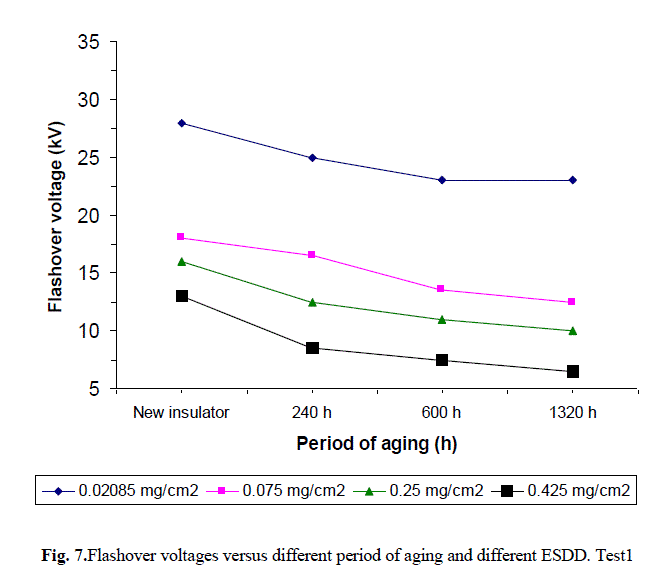The output waveform of inverter output as shown in figure 10a.When manual switch is connect directly to the output of inverter, there is a dc voltage of 1 V appears at output. After switch connected to PI, the output voltage is minimized as shown in figure 10b.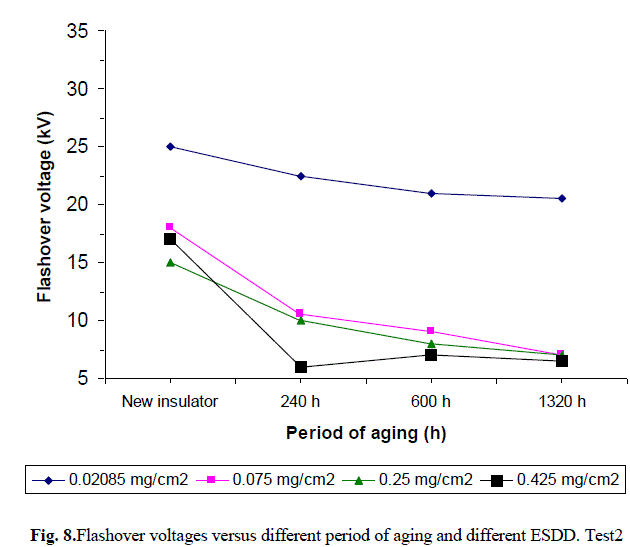The THD value can be obtained by using FFT analysis, as shown in figure 11 and frequency spectrum of proposed system as shown in figure 12. Both are clearly shows harmonics are minimized and THD obtained is 4.24% , complies with the limit imposed by the standards.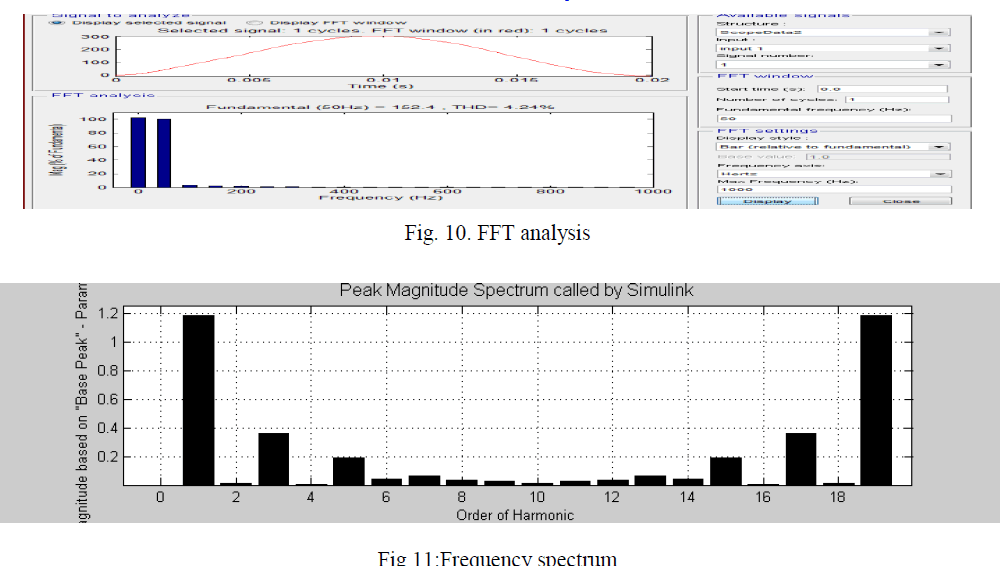### VI.CONCLUSION

A novel approach to harmonic minimization method for single phase inverter established on mirror injection of harmonic and elimination of dc principle has been proposed. The following conclusions can be drawn after the imaginary analysis and the experimental results reported in this paper the proposed system minimizes the harmonics generated by the switching operation as well as harmonics generated by dc nature of the source and external point i.e. grid. Improvement of THD, through the cancellation of low-order harmonics and minimization of dc , so that the output voltage complies with the limit imposed by the standards, without the use of a filter.

### References

 Yong-Won Cho, Jung-Min Kwon, and Bong-Hwan Kwon,” Single Power-Conversion AC–DC Converter With High Power Factor and High Efficiency”, ieee transactions on power electronics, vol. 29, no. 9, PP.4797 4806,september 2014.

 B. Singh, B. N. Singh, A. Chandra, K. Al-Haddad, A. Pandey, and D. P. Kothari, “A review of single-phase improved power quality AC– DC converters,” IEEE Trans. Ind. Electron., vol. 50, no. 5, pp. 962–981, Oct. 2003.

 B. Singh, B. N. Singh, A. Chandra, K. Al-Haddad, A. Pandey, andD. P. Kothari, “A review of three-phase improved power quality ac– dcconverters,” IEEE Trans. Ind. Electron., vol. 51, no. 3, pp. 641–660, Jun.2004.

 H. S. Kim, M. H. Ryu, J. W. Baek, and J. H. Jung, “High-efficiency isolatedbidirectional AC–DC converter for a DC distribution system,” IEEETrans. Power Electron., vol. 28, no. 4, pp. 1642–1654, Apr. 2013.

 M. Arias, D. G. Lamar, J. Sebastian, D. Balocco, and A. A. Diallo, “HighefficiencyLED driver without electrolytic capacitor for street lighting,”IEEE Trans. Ind. Appl., vol. 49, no. 1, pp. 127–137, Jan./Feb. 2013.

 B. Tamyurek and D. A. Torrey, “A three-phase unity power factor singlestageAC–DC converter Based on an interleaved flyback topology,” IEEETrans. Power Electron., vol. 26, no. 1, pp. 308–318, Jan. 2011.

 S. C.Moon, G. B. Koo, and G. W.Moon, “A new control method of interleavedsingle-stage flyback AC–DC converter for outdoor LED lightingsystems,” IEEE Trans. Power Electron., vol. 28, no. 8, pp. 4051–4062, Aug. 2013.

 M. Narimani and G. Moschopoulos, “A new single-phase single-stagethree-level power factor correctionAC–DCconverter,” IEEE Trans. PowerElectron., vol. 27, no. 6, pp. 2888–2899, Jun. 2012.

 C. Y. Oh, D. H. Kim, D. G. Woo, W. Y. Sung, Y. S. Kim, and B. K. Lee,“A high-efficient nonisolated single-stage on-board battery charger forelectric vehicles,” IEEE Trans. Power Electron., vol. 28, no. 12, pp. 5746–5757, Dec. 2013.